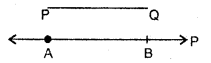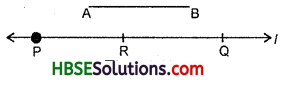# HBSE 6th Class Maths Solutions Chapter 14 Practical Geometry Ex 14.3

Haryana State Board HBSE 6th Class Maths Solutions Chapter 14 Practical Geometry Ex 14.3 Textbook Exercise Questions and Answers.

## 14.3

Question 1.
Draw any line segment $$\overline{\mathrm{PQ}}$$. Without measuring $$\overline{\mathrm{PQ}}$$ construct a copy of $$\overline{\mathrm{PQ}}$$.
Solution:
(i) Given $$\overline{\mathrm{PQ}}$$ whose length is not known.(ii) Fix the compasses pointer on P and the pencil end on Q. The opening of the instru-ment now gives the length of $$\overline{\mathrm{PQ}}$$.
(iii) Draw any line ‘l’. Choose a point A on ‘l’. Without changing the compasses setting, place the pointer on A.
(iv) Strike an arc that cuts ‘l’ at a point, say, B. Now $$\overline{\mathrm{AB}}$$ is a copy of $$\overline{\mathrm{PQ}}$$.Question 2.
Given some line segment $$\overline{\mathrm{AB}}$$, whose length you do not know, construct $$\overline{\mathrm{PQ}}$$ such that the length of $$\overline{\mathrm{PQ}}$$ is twice that of $$\overline{\mathrm{AB}}$$.
Solution:
(i) Given $$\overline{\mathrm{AB}}$$ whose length is not known.(ii) Fix the compasses pointer on A and the pencils end on B. The opening instru¬ment now gives the length of $$\overline{\mathrm{AB}}$$.
(iii) Draw any line ‘l’. Choose a point P on ‘l’. Without changing the compasses setting, place the pointer on P.
(iv) Strike an arc that cuts ‘l’ at a point R.
(v) Now, place the pointer on R and without changing the compasses setting, strike another arc that cuts ‘l’ at a point Q,
(vi) Thus, $$\overline{\mathrm{PQ}}$$ is the required line segment whose length is twice that of AB.